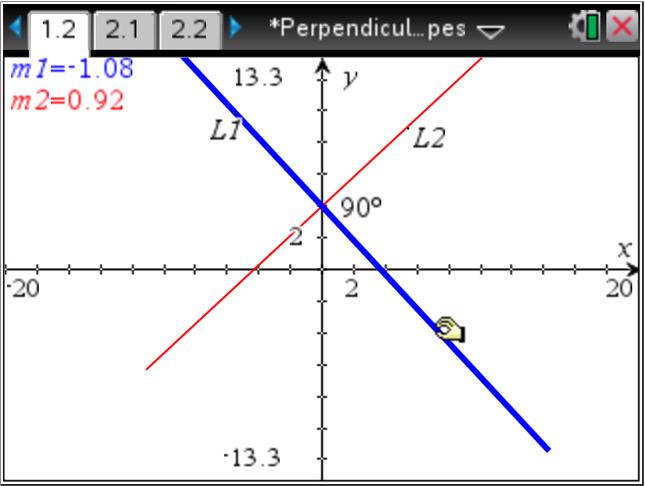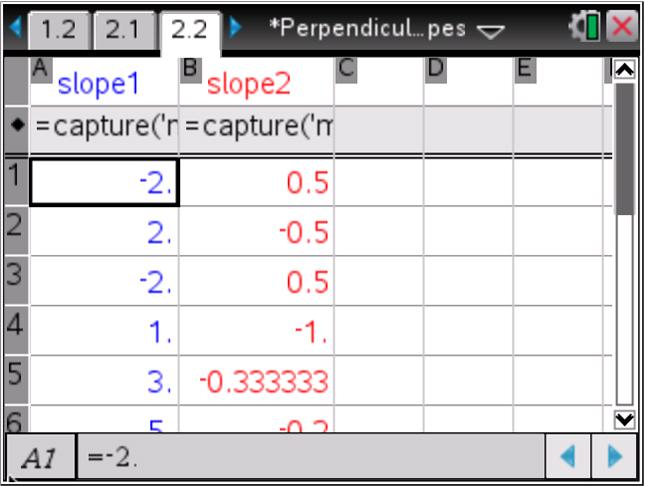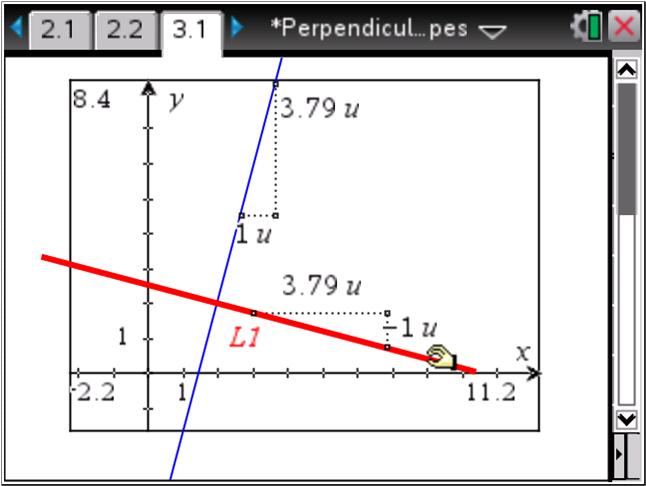# Activities

••• ##### Subject Area

• Math: Algebra I: Linear Functions

• ##### Author9-12

45 Minutes

• ##### Device
•TI-Nspire™ CX/CX II
•TI-Nspire™ CX CAS/CX II CAS
• TI-Nspire™
• TI-Nspire™ CAS
• ##### Software

TI-Nspire™
TI-Nspire™ CAS

3.2

## Perpendicular Slopes

#### Activity Overview

Students investigate the 'negative reciprocal' relationship between the slopes of perpendicular lines. The final phase of the activity is appropriate for more advanced students as they are led through an algebraic proof of the relationship. Optional geometric activities (problems 5 and 6 of the .tns file) use the result to verify that (1) the radius of a circle and its tangent line are perpendicular and (2) a triangle inscribed in a circle with the diameter as one side is a right triangle.

#### Key Steps

•Students will first examine the relationship between the slopes of perpendicular lines by rotating line L1 and comparing the slopes m1 and m2.

•After choosing several different values of slopes on the graph, students can view the results in the spreadsheet on page 2.2. They will determine an algebraic formula that relates m1 and m2. Students can test the formula in the gray formula cell in Column C.

•Students are provided another look at the relationship between the slopes of two perpendicular lines, represented by triangles with a hypotenuse on each line and one leg of length 1.